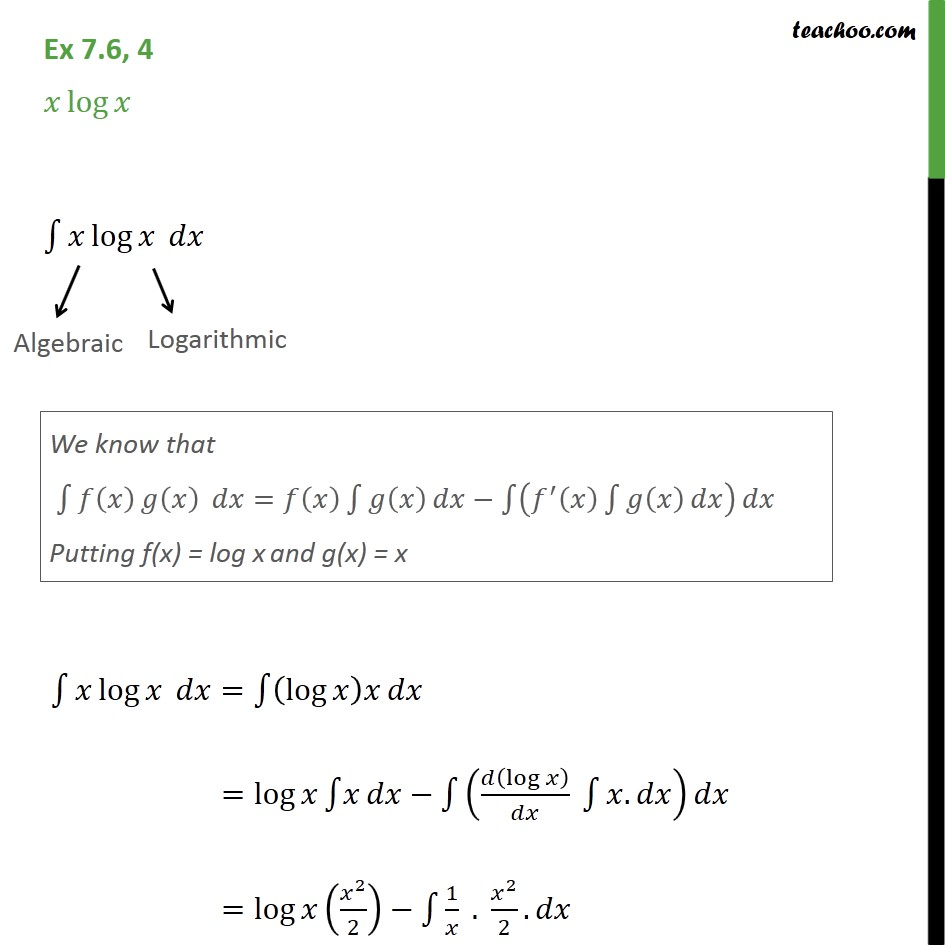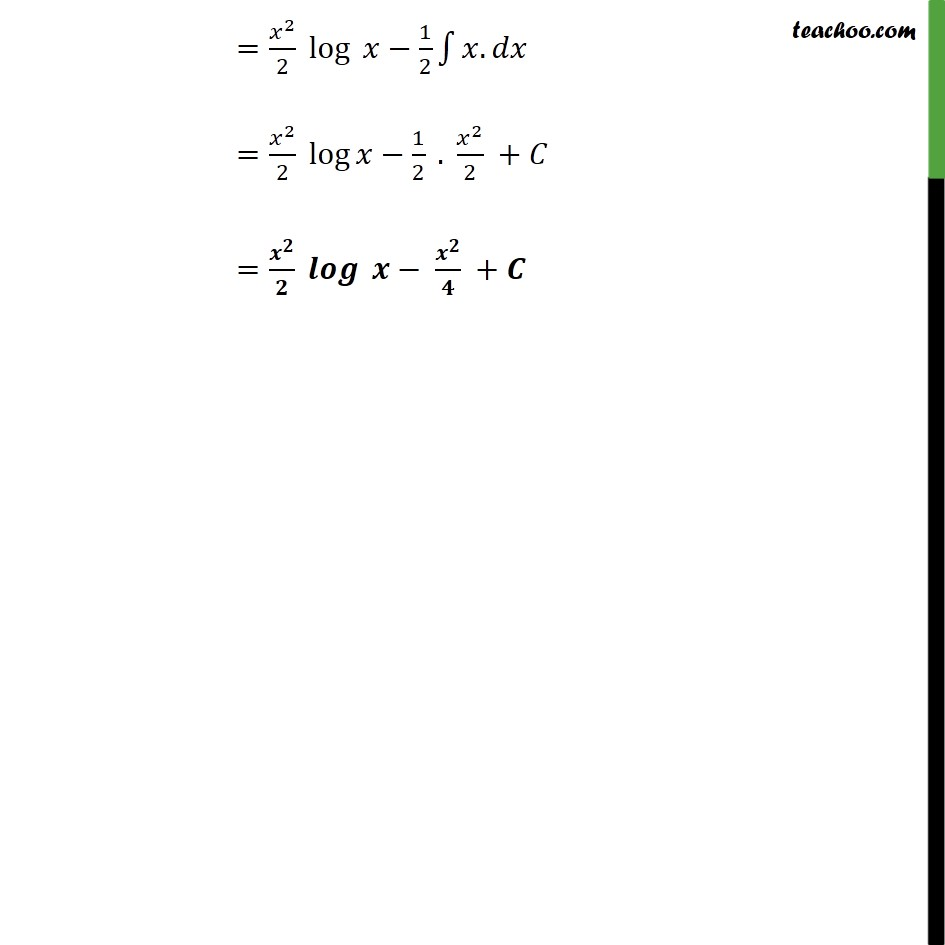Integration Full Chapter Explained - https://you.tube/Integration-Class-121. Chapter 7 Class 12 Integrals
2. Serial order wise
3. Ex 7.6

Transcript

Ex 7.6, 4 log 1 log 1 log = 1 (log ) =log 1 1 ( (log )/ 1 . ) =log ( ^2/2) 1 1/ . ^2/2. = ^2/2 log 1/2 1 . = ^2/2 log 1/2 . ^2/2 + = ^ / ^ / + " "

Ex 7.6

Chapter 7 Class 12 Integrals
Serial order wise